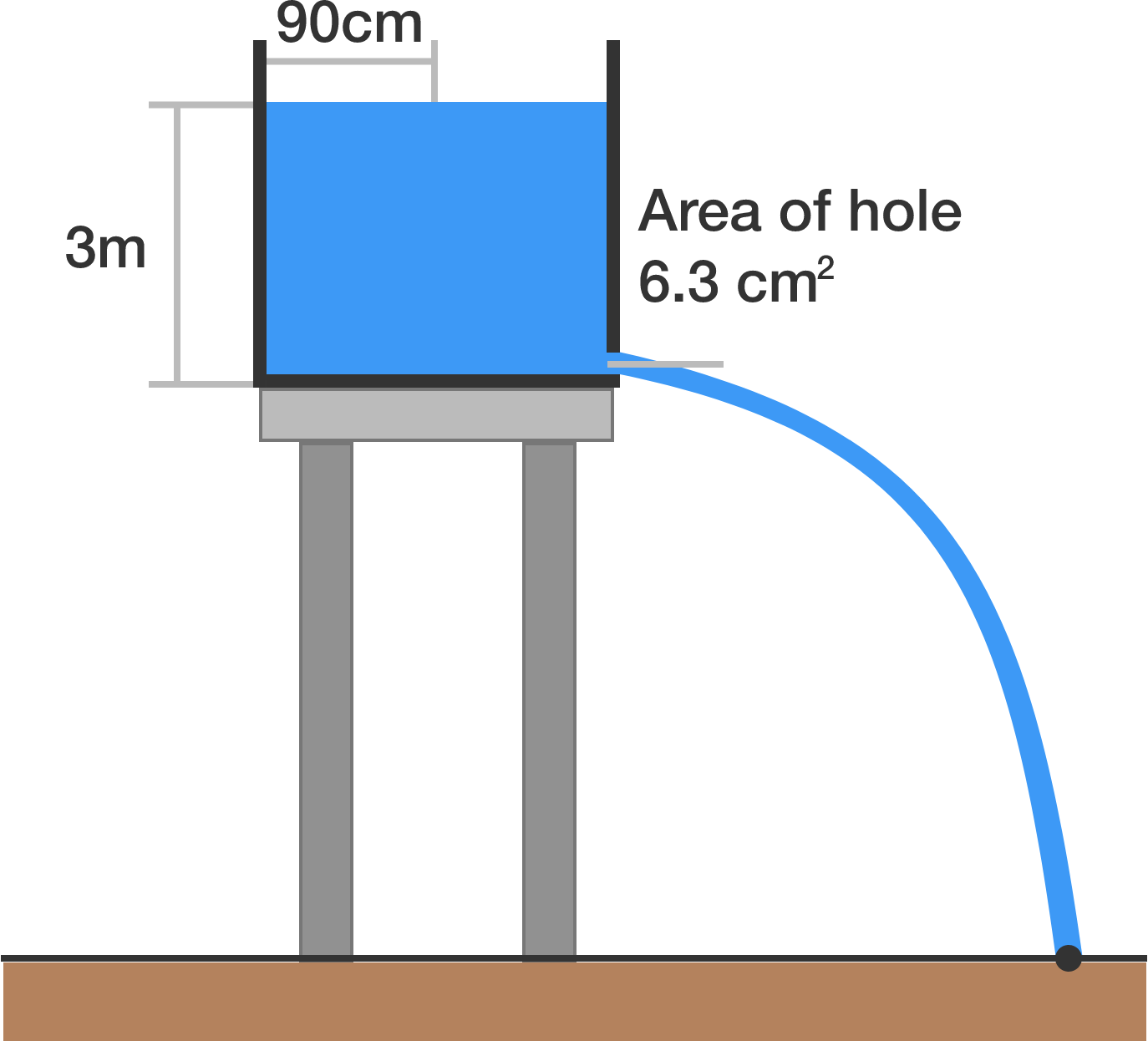# Empty the vesselA cylindrical vessel with radius $90 \text{ cm}$ filled with 3-meter deep water is sitting on a tower, as shown in the diagram. The vessel has a hole on the bottom with a cross-sectional area of $6.3 \text{ cm}^2$.

How long (in minutes) will it take before the vessel is completely drained through the hole?

Assume that the gravitational acceleration is $g = 9.8 \text{ m/s}^2.$

×